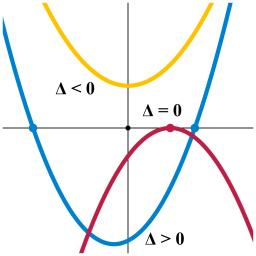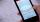# Discriminant

Determine the discriminant of the equation:

$7{x}^{2}-x+7=1$

Correct result:

D =  -167

#### Solution:

$D={b}^{2}-4ac=\left(-1{\right)}^{2}-4\cdot 7\cdot \left(7-1\right)=-167$We would be pleased if you find an error in the word problem, spelling mistakes, or inaccuracies and send it to us. Thank you!Tips to related online calculators

## Next similar math problems:

• SeatsSeats in the sport hall are organized so that each subsequent row has five more seats. First has 10 seats. How many seats are: a) in the eighth row b) in the eighteenth row
• CombinationsFrom how many elements we can create 990 combinations 2nd class without repeating?
• Roots countSubstitute the numbers/0,1,2,3/into the equation as x: (x - 1) (x - 3) (x + 1) = 0 Which of them is its solution? Is there another number that solves this equation?
• Function 3Function f(x)=a(x-r)(x-s) the graph of the function has x- intercept at (-4, 0) and (2, 0) and passes through the point (-2,-8). Find constant a, r, s.
• Expressions 3If k(x+6)= 4x2 + 20, what is k(10)=?
• ProductThe product of two consecutive odd numbers is 8463. What are this numbers?
• Find the 20Find the product and the sum of the roots of x2 + 3x - 9 = 0
• CombinationsHow many elements can form six times more combinations fourth class than combination of the second class?Quadratic equation ? has roots x1 = -26 and x2 = -86. Calculate the coefficients b and c.If 5x + x² > 100, then x is not
• Solve 3Solve quadratic equation: (6n+1) (4n-1) = 3n2
• EquationEquation ? has one root x1 = 8. Determine the coefficient b and the second root x2.
• AlgebraX+y=5, find xy (find the product of x and y if x+y = 5)Which of the points belong function f:y= 2x2- 3x + 1 : A(-2, 15) B (3,10) C (1,4)Determine the quadratic equation absolute coefficient q, that the equation has a real double root and the root x calculate: ?Find the roots of the quadratic equation: 3x2-4x + (-4) = 0.Determine the number of items when the count of variations of fourth class without repeating is 600 times larger than the count of variations of second class without repetition.